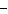# Algebra 2 Absolute Value Quiz

Multiple Choice
Identify the choice that best completes the statement or answers the question.
Solve the given equation. Check your solution.

1.1 | = 23
 a. {24, –22} b. {24, 29} c. {–24, –22} d. {24, 22}

2.

20| 2+ 5 | = 24
 a. {–1.9, –3.1} b. {–1.9, 3.1} c. {9.5, –3.1} d. {1.9, –3.1}

3.

112| 2+ 5 | = 116
 a. {–1.98, –3.02} b. {1.98, –3.02} c. {55.5, –3.02} d. {–1.98, 3.02}

4.

m – 6 | = 27
 a. {–33, –21} b. {33, 21} c. {33, 29} d. {33, –21}

5.

Evaluate the given expression if = 61.
| -3|
 a. 183 b. 438 c. 61 d. –183

6.

Evaluate the given expression if k = –48.
5| k + 10 | – | 4|
 a. 382 b. 2 c. –10 d. –2

1-4. For any real numbers and b, where0, if | a | = b, then a = b, orb.